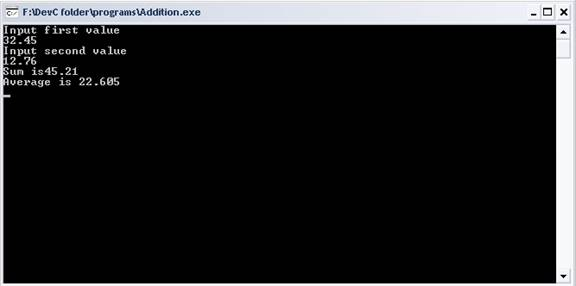### Input values using cin operator

Before reading the tutorial, you should be familiar with variables and data types, find about them in tutorial on Variables and Data Types.

A computer program is an interactive program which takes values from user. To input values, C++ uses the Stan  Standard Input Stream cin operator. It is same as cout operator and is a predefined object in C++ which is normally attached to keyboard but can be attached to any other input device such as printer. Following example illustrates use of cin operator, where we input two values using cin operator and print sum and average of those values.

 # include int main () { float var1, var2, sum, avg; std::cout << "Input first value" << endl; std::cin >> var1; std::cout << "Input second value" << endl; std::cin >> var2; sum = var1+ var2; avg = sum / 2; std::cout << "Sum is" << sum << endl; std::cout << "Average is " << avg << endl; return 0; } Input first Value 32.45 Input second value 12.76 Sum is 45.21 Average is 22.605

Lets say we in input the values 32.45 and 12.76, so the program will output sum as  45.21 and average as 22.605.

The program is similar to our first C++ program except that it declares variables var1, var2, sum and avg of type float to take values from user and to compute sum and average. Statement

float var1, var2, sum, avg; does this.

Next, we prompt the user to input values and take values using the cin operator. When the user inputs values and hits enter, then the value entered by user is collected by the cout object and send to the variable var2. Similarly, we input second value and send it to var2. Statements

std::cin << var1;

std::cin << var2; accomplish this.

<< is a stream extraction operator and its work is to extract values from operand on its left hand and assign it to operand on its right hand. In this case, it takes value from cin and assigns to variables var1 and var2.

Statements

sum = var1 + var2;

avg =  sum/2;

compute sum and average of the two values. First statement computes sum of values in var1 and var2 and assigns it to variable sum.

Second statement computes average by dividing sum by 2 and assigning the result to avg variable. These statements make use of arithmetic addition (+) operator. It will be discussed later in tutorial on Operators in C++.

Next statement

std::cout << "Sum is" << sum << endl;

prints the string and "Sum is" and then prints the value of sum variable and points the cursor the next line in the screen. Here, you can use multiple stream insertion operators (<<) in a single statement. This is known as cascading of operators. Similarly,

std::cout << "Average is " << avg << endl;

prints string "Average is " and then prints values of avg variable and points the cursor to the next line in the screen.

Finally the last statement returns value 0 to operating systems indicating that the program completed successfully.

Here is the screenshot of program when run in DevC++ compiler.Here is the output of the program.Go to the previous lesson or proceed to the next lesson